### Home > A2C > Chapter 11 > Lesson 11.2.4 > Problem11-87

11-87.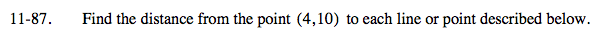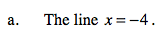Find the distance between the two x values.

8 units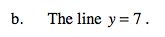See part (a).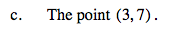Use the distance formula.

$d=\sqrt{(4-3)^{2}+(10-7)^{2}}$

$\sqrt{10}\text{ units}$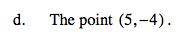See part (c).